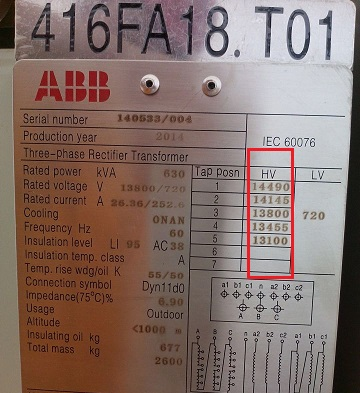Technology Literature

# What is the rated turns ratio, actual turns ratio, standard and non-standard turns ratio ?

Transformer ratio K (ie voltage ratio) is the ratio of the high-voltage winding voltage U1 to the low-voltage winding voltage U2 under the no-load condition of the transformer. The transformation ratio is divided into four types: rated transformation ratio, actual transformation ratio, standard transformation ratio and non-standard transformation ratio. What is the difference between these transformation ratios?

Rated turns ratio

Under the no-load condition of the transformer, the ratio of the rated high-voltage winding voltage U1 to the low-voltage winding voltage U2 can also be said to be the ratio of the primary voltage to the secondary voltage of the transformer.

Actual ratio

Center tap ratio. Some transformers have different taps on the high-voltage side, so the transformer has the concept of rated transformation ratio and actual transformation ratio, such as the following figureStandard turns ratio

When the input of each tap is 0, the instrument does not automatically judge the tap position, and always takes the input high and low pressure ratio as the standard transformation ratio.

Non-standard turns ratio

The transformation ratio that does not belong to the national standard, such as 10000/27, or some special requirements' voltage, is realized by the non-standard transformation ratio.

All Kingrun Testers will be Delivered by International Air Express for Free!